2014-10-02

What the Heck is Amortized Constant Time Anyway?

Effective use of the Scala Collections Library benefits from a solid understanding of the performance characteristics of the operations on a collection. In this regard, the web page Scala Collections Performance Characteristics is a valuable resource, as it summarizes the performance characteristics of various collections in easy to read charts. We see two interesting entries on these charts: effective constant time and amortized constant time. Just what do these terms mean, and how does it affect us as Scala programmers?

In this article, we will discuss amortized constant time - a performance characteristic for mutable collections such as Java's ArrayList, HashMap and HashSet. We'll address effective constant time - seen in immutable collections such as Scala's Vector, HashMap and HashSet - in a later essay.

Constant Time and Linear Time

Let's start out by reviewing two more straightforward measures of time complexity: constant time and linear time. When we do time complexity analysis, we are interested in how the performance of an algorithm changes with respect to its input size. In regards to Scala collections, and collections in general, the algorithms are the operations on the collection itself, and the input size is the size of the collection. Time complexity analysis is important because, while an operation may perform well when the size of the collection is small, we may run into performance problems when handling larger collections. For example, our code may run very fast on the test data that we use in our automated testing and our example systems, but perform badly in a production environment.

In the best case, the performance is independent of the input size. We can perform updates and lookups on our collection, and performance does not degrade no matter how large our collections get. In this case, we can find a constant, c, so that for any input size, n, the operation completes in time less than or equal to c. (Note that our constant c, is a quantity of time, and should have a time unit, such as milliseconds.) We let t(n) be a function denoting how much time it takes for the operation to complete on input size n. If we can find a c such that for all n, the following equation holds:

Then we know that the operation is constant time. Using Big O notation, we say that the operation is O(1). Let's take note that we are not saying anything about what that constant c is. It could be small, or it could be quite large. You could have two different constant time operations, one of which performs much better than the other. But in time complexity analysis, they are viewed as equivalent. Here, we are strictly interested in how the operation performs as our input size increases. This is a limited view on software and systems performance in general, but it helps us isolate a key scalability concern.

Another common time complexity we encounter is linear time. Here, the amount of time it takes for the operation to complete grows with the size of the input. Roughly speaking, if 100 elements take 100 milliseconds, then 1000 elements should take about 1000 milliseconds, and so on. Here, we choose two constants, b and c, so that for all n, the following equation holds:

Note once again that both constants b and c have units time. We can consider b to represent how long it takes the operation to process each input element, and c to represent an operation overhead - the work that must be performed by the operation regardless of the input size. This is a linear time operation, we we express this in Big O notation as O(n).

There are many other kinds of time complexity worth studying, such as logarithmic, O(log n), quadratic, O(n2), and exponential, O(2n). But we focus on constant time and linear time here because, as we will see, amortized constant time operations are built from constant time and linear time operations.

An Abstract Data Type for an Efficient Mutable Sequence

Let's suppose we needed to implement an efficient, mutable sequence. A mutable sequence is a collection with which we can look up elements according to an integral index value, overwrite elements stored at a given index, and add or remove elements at the end position. In a collections library, a sequence would have many more operations, but we can focus on these four as a core set. By "efficient", we mean that these operations should occur in constant time, or a close approximation to constant time. We can describe such an abstract data type, or ADT, in a trait such as this:

The @throws annotations in the ADT define the boundaries of usage. For instance, you can't unappend from an empty buffer. You also cannot access into the sequence with an index that goes outside of the sequence's current bounds. Because this applies to update as well as apply, the only way to extend the current bounds of the sequence with with append.

Of course, we would never actually need to implement such a thing, as we will find one included in the standard library of nearly every modern programming language that allows for mutable data. For instance, in Java we would use java.util.ArrayList. In Scala, we use scala.collection.mutable.ArrayBuffer. But it's worth having a clear understanding of how these things work, so we can gauge the performance implications of using these library classes in our code.

A Naive Buffer Implementation

When considering an implementation for this ADT, we take note that access into the sequence is by index, and this access has to be fast. Our instincts tell us to use an array - a contiguous block of memory used to store multiple elements of the same type. Access by index is fast, as the memory location for any particular index can be computed based on the location of the array, the size of the array elements, and the index itself. Because our sequence can change in size, we only use a portion of the array at any given time, and we need to keep track of the current size of the sequence, or how much of the array is used. To get a sense of what we are talking about, here's a picture of a data structure we might use to hold the array, and keep track of the size of the sequence: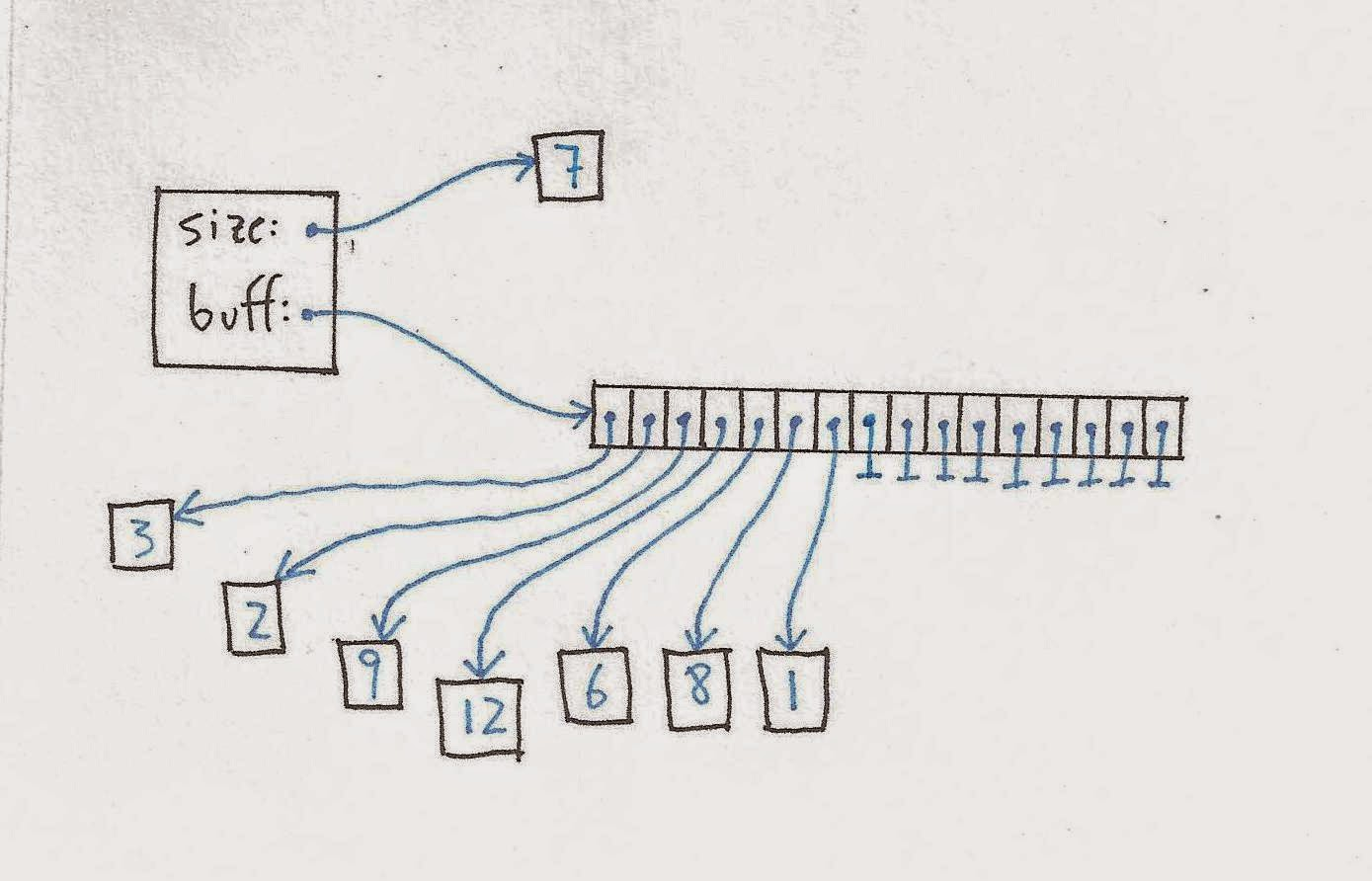Figure 1: a naive array buffer with size 7
The example stores a sequence of integers, but we could store elements of any type in this fashion. All the array elements that are not used to store elements of the buffer contain null.

When we append a new integer, we simply increment the size, and update the array element at the end of our buffer. For instance, if we call append(7) to the above example, we end up with this:Figure 2: a naive array buffer with size 8
If we then call unappend(), we decrement the size, and update the array element at the end of the buffer to null. This last bit is important to avoid memory leaks. When we do so, we end up back in the the example of figure 1.

Now that we have the basic idea, we can implement the Buffer trait as follows:

Looking over this code, we can feel satisfied that all four NaiveArrayBuffer operations are O(1). For each operation, we can choose a constant time value c, so that the operation will always complete in time less than c, regardless of the current size of the buffer. We know this because each step in each of the operations, including the array operations, are fixed time operations.

You've probably seen the fatal flaw in our NaiveArrayBuffer already: our sequence can only store as many elements as the array can hold! If we try to call append when the size of the sequence is 16, the array update operation itself will throw an IndexOutOfBoundsException. One not so satisfactory approach to remedying this is to simply allocate an array that is going to be large enough for our needs. I won't digress too far on this, but I'd like to mention that we used to do this kind of thing in C all the time, back in the day: just pick a size that you are sure is going to be big enough. This is not as bad as you might think, since even if you allocate an array with a million elements, that array only exists in virtual memory until it is actually used. Once used, the array will only be allocated to physical memory on a page by page basis, and back then at least, pages were 4KB. So the actual physical memory used would be bounded by the amount of the array actually used, with an overhead of 4KB or less.

But you probably won't see this kind of behavior in a virtual machine such as the JVM. For one thing, the JVM assures that a newly initialized array contains all zeroes or nulls, so it must pass over the entire array, writing zeroes as it goes. This means the entire array is "used" before we even get our hands on it! In any event, we definitely want a more robust approach, so we have to figure out what to do when our array fills up.

An Expanding Array Buffer

To solve the problem of our array running out of space, we simply allocate a new, larger array, copy the contents over, and discard the old array. The new implementation looks just like our NaiveArrayBuffer, with the append method modified to expand when needed:

But what can we say about the time complexity of this new sequence implementation? The unappend, update, and apply are unchanged, and still perform in constant time. Most of the time, append will still be O(1), but when the buffer is expanded, the performance will be linear, or O(n). We know this to be true because both creating the new array, and copying over the contents, are O(n) operations themselves.

Let's look at that in a little more detail, to help get a feel for how time complexity analysis works. The two array operations, new and copy, have their own constants b and c. Let's subscript them with n and c, so we can tell them apart. If tn(n) and tc(n) compute how long these operations take to complete, then we can say:

Let's represent the remaining parts of the append operation with function tr(n). This work completes in constant time, so we have:

Now let's put it all together and see what we can say about te(n), the time it takes for an append operation to complete when the array needs to be replaced. Note that when we create the new array, it is twice as big as the initial size of the collection. We have:

Choosing (2bn + bc) for be, and (2cn + cc + cr) for ce, we get

So when the buffer must expand, the append operation is of linear time complexity, or O(n). But we only get this linear behavior once in a while. Normally the append operation is O(1). Are we stuck with considering this a linear operation? How can we combine the varying behaviors of this method to get a more precise picture of it's overall behavior?

A Review in Charts

Let's review the three time complexities we've encountered so far - constant time, linear time, and the varying behavior of ExpandingArrayBuffer.append - in chart format, to get a better understanding of what is going on. We recall that a constant time operation is bounded by some constant c. It doesn't really matter what the constant is, so we'll just use 7. In the following bar chart, the input size, n, is plotted along the x-axis, and the time to complete the operation, t(n), is plotted along the y-axis. Regardless of the input size, the operation always takes the same amount of time to complete: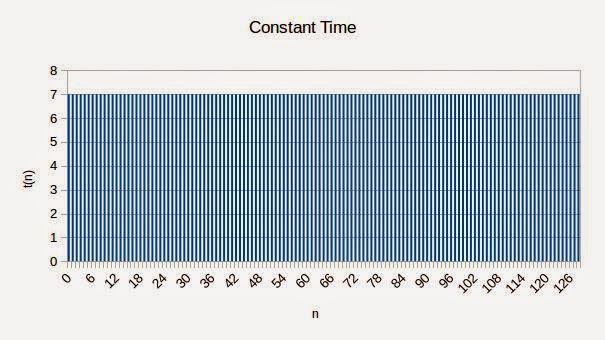Figure 3: Constant Time Bar Chart

Now let's turn to linear time. A linear time operation completes in no less time than b · n + c, for some constants b and c. Again, the specific values of the constants do not matter. We will use 3 and 27 for the sake of this example. Although the bar chart plots discrete values, you can easily visualize a line that goes through the tops of all the bars: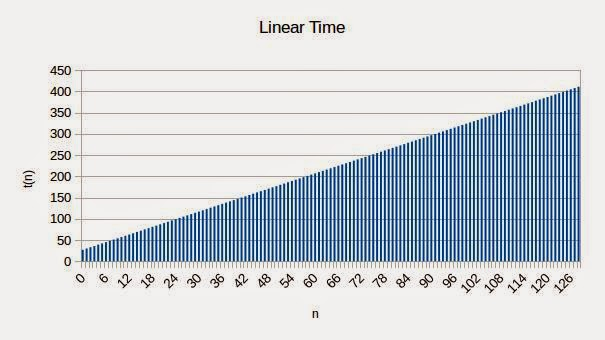Figure 4: Linear Time Bar Chart
Now let's look at our ExpandingArrayBuffer.append operation. In this chart, we've modified things slightly so that the expansion behavior occurs for every n that is a power of two, instead of kicking in when we hit size 16. In theory, we could change the value of StartSize to 1 to produce this result. I've made this adjustment so that we can more easily see the trends of the buffer-expanding appends - both their spacing along the x-axis, as well as the linear trend you can see by visualizing a line between the tops of these bars. Here is the chart: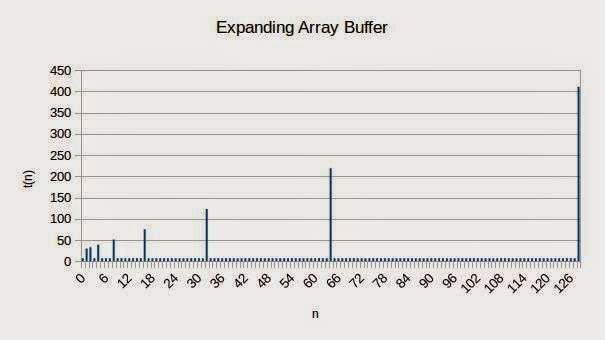Figure 5: Expanding Array Buffer Time Complexity
We can see from the chart that while the expanding appends grow linearly, their spacing gets farther and farther apart, suggesting that these numbers play a less significant role than the constant time operations. We can get imaginative, and picture pushing these bars over, like knocking over dominoes. Once we get past the first two or three tall bars, the dominoes are spaced too far apart to knock each other over. This is suggestive of an overall constant time operation, if we are willing to consider these occasional costs as spread over a series of append operations. And this is precisely the intuition behind amortized constant time, which will we discuss next.

Amortized Time Complexity

When we're appending to our sequence, we do not care so much about how long the individual append operations take. Rather, we are interested in how long an append operation takes on average. Consider building up a sequence of a million elements using the append operation. It really doesn't matter to us if 10 or 20 of those million operations take a very long time. What matters is the amount of time it takes to do all the appends. This is still a function of n, the ultimate size of the sequence, but it is different than the t(n) function that we have looked at so far, which measures the time taken by a single append operation. Let's call this new function amortized time, or a(n), and define it as the average of all t(i) for 0 ≤ i ≤ n:

Let's look at the three time functions we considered in the previous section. First, a constant time operation takes the same amount of time for each iteration, regardless of n. Clearly, the average of n + 1 instances of c will be c, so a(n) = t(n) for all constant time operations. If we were to plot this function on a bar chart, the result would be exactly the same as the bar chart shown in figure 3.

Let's quickly consider a linear time function as well. If an operation has a linear time complexity, then it should have an amortized linear time complexity as well. The following chart plots a(n) for the t(n) plotted in figure 3:Figure 6: Linear Time, Amortized Bar Chart
This function is clearly linear as well. We'll save doing out the math to show that this function is linear as an exercise to the reader.

Now let's consider our ExpandingArrayBuffer.append operation. Here is the chart:Figure 7: Expanding Array Buffer Amortized Time Complexity
We can see from the chart that the function has a clear upper bound of around 23 or 24 when n is 2, indicating that this function indeed has a constant upper bound. Each time we hit an append operation that allocates a larger array, the amortized time jumps up, but each such jump hits a lower high than the previous. The last such append operation shown, when n is 128, has a(n) at just over 13.

The charts in this section and the previous section were all created using LibreOffice Calc. Please feel free to download the spreadsheet, in either ODS or XLSX format, and play around with it. You might fiddle with the constants and see how your changes effect the charts.

Deriving the Time Complexity for the Append Operation

The chart in figure 7 is a pretty convincing demonstration that the append operation performs in amortized constant time. But let's keep going and see if we can derive this mathematically. What we want to show is that there is some constant c for which a(n) is less than or equal to c for all n. Let's start by breaking up the range of n into groups. In each group, we want to include 1 buffer-expanding append, plus all of the append operations that occur before that, up to the previous buffer-expanding append. For instance, one group would include appends for 64 < n ≤ 128. If we can show that for every such group, the average value of t(n) is bounded by the same constant, then the average of the whole series is also bounded by that same constant.

First, we define a variable i as the log2(n). Note that i is only defined where n is a power of 2:

Now we can define a function r(i) that takes the average of the performance times across such a group. There are n/2 values being averaged, which explains the 2/n factor at the front:

Let's define functions for the non-expanding append - an O(1) operation - and the expanding append - an O(n) operation:

Now out of the n/2 operations being averaged, all but one is governed by tn(n). The remaining operation is governed by te(n). So we can expand the summation in equation 9 as follows. Can you justify each step in the derivation?

This shows that r(i) is always bounded by the constant cn + 2be + ce, regardless of i. We now have a series of groups of numbers, with the average value for each group bounded by the same constant. Intuitively, we know the average for the whole series is bounded by that constant as well. It could use a proof, but we will leave that as another exercise to the reader.

A Retracting Array Buffer

Our ExpandingArrayBuffer has a fatal flaw that you might have noticed earlier. We expand the buffer to allow for the sequence to grow, but sequences can also shrink via the unappend operation! What if we called append 1000 times, and then called unappend 990 times? We would be left with an array of size 1024, with only the first 10 slots of the array in use. This may be fine for many use cases, but for a data structure provided by a collections library, we need to be more thoughtful about our memory use, and reduce the size of the buffer when the sequence shrinks. As with expanding the array buffer, this is an O(n) operation, so we don't want to retract on every unappend operation. As an initial, naive attempt, we could retract whenever the buffer becomes half empty. We also retract so that the buffer and the array have the same size:

Now we retract our array so that we never use more than twice the space as the actual size of the buffer. But our initial solution has a serious flaw. Suppose the current size of our buffer was 16, (or any other power of two), and we proceeded to issue a number of append and unappend operations in pairs. The first append operation would expand the array to 32, and the following unappend would retract it to 16. And the same thing would happen for every subsequent pair of append/unappend operations. Each expansion or contraction is an O(n) operation, so we lose our amortized constant time behavior when we allow for the interleaving append and unappend operations.

Two potential solutions are strongly hinted at in the code presented above. First, we could choose to retract the buffer when the array is less than half full. For instance, we could retract when the buffer was one quarter full. The downside to this is that we will allow for more wasted space. A second approach would be to not retract it so much, leaving a little "buffer" room for upcoming append events. For instance, we could set the RetractionRatio to 1.5. In this case, when an unappend operation reduces the size of the sequence from 17 to 16, the 32-element array will be replaced with a 24-element array, and the following append will not cause another expansion.

We could likewise tweak the ExpansionRatio to get a similar effect. If the ExpansionRatio were 3, and the RetractionTriggerRatio were 2, we would also avoid thrashing the buffer when interleaving append and unappend events. There is an interplay between all these constants, involving tradeoffs between the amount of wasted space we are willing to accept, and the frequency of the more expensive operations involving resizing the array.

Hash Sets and Hash Maps

When discussing amortized constant time, it's important to mention the hash table based implementations of sets and maps. These are the most commonly encountered implementations of mutable sets and maps, and include java.util.HashSet, java.util.HashMap, scala.collection.mutable.HashSet, and scala.collection.mutable.HashMap. They display a very similar expanding/contracting behavior as the mutable sequence we discussed above. Consequently, their add and remove operations display the same amortized constant time behavior.

It's beyond the scope of this essay to go into these collections in detail, but I thought it would be helpful to present another example of operations with this kind of behavior, so we don't walk away with the impression that amortized constant time is all about mutable indexed sequences. If you want to learn about these in more detail, there are a number of good sources, including the wiki page for hash table.

Let's look briefly at the anatomy of a hash set. Hash maps follow a very similar structure, except that they store key-value pairs instead of individual elements.

Similar to the ArrayBuffer class we discussed above, a hash set has local variables to keep track of its current size, and an array in which to hold the contents of the set. Unlike ArrayBuffers, the elements are not accessed by a simple integer index. Instead, elements are accessed using hashCode and equals (or some non-Java/Scala equivalent of the two). The slots in the array are generally referred to as buckets, or hash buckets. Each bucket contains a linked list - ideally a very short linked list - of the set elements that belong in that bucket. How do we know which bucket an element belongs to? We take its hashCode, modulo the length of the array. (In other words, divide the hashCode by the length of the array, and take the remainder.)

Now that's quite a mouthful. Let's see if a picture will help. We'll consider the set of integers {21, 29, 36, 42, 54, 66, 71}. It has size 7, and its elements will be stored in an array of size 10. Conveniently enough, the hashCode of an integer is itself. Taking the modulo 10 of the hashCode will yield the least significant digit of the integer. Here is an example of what such a hash set might look like: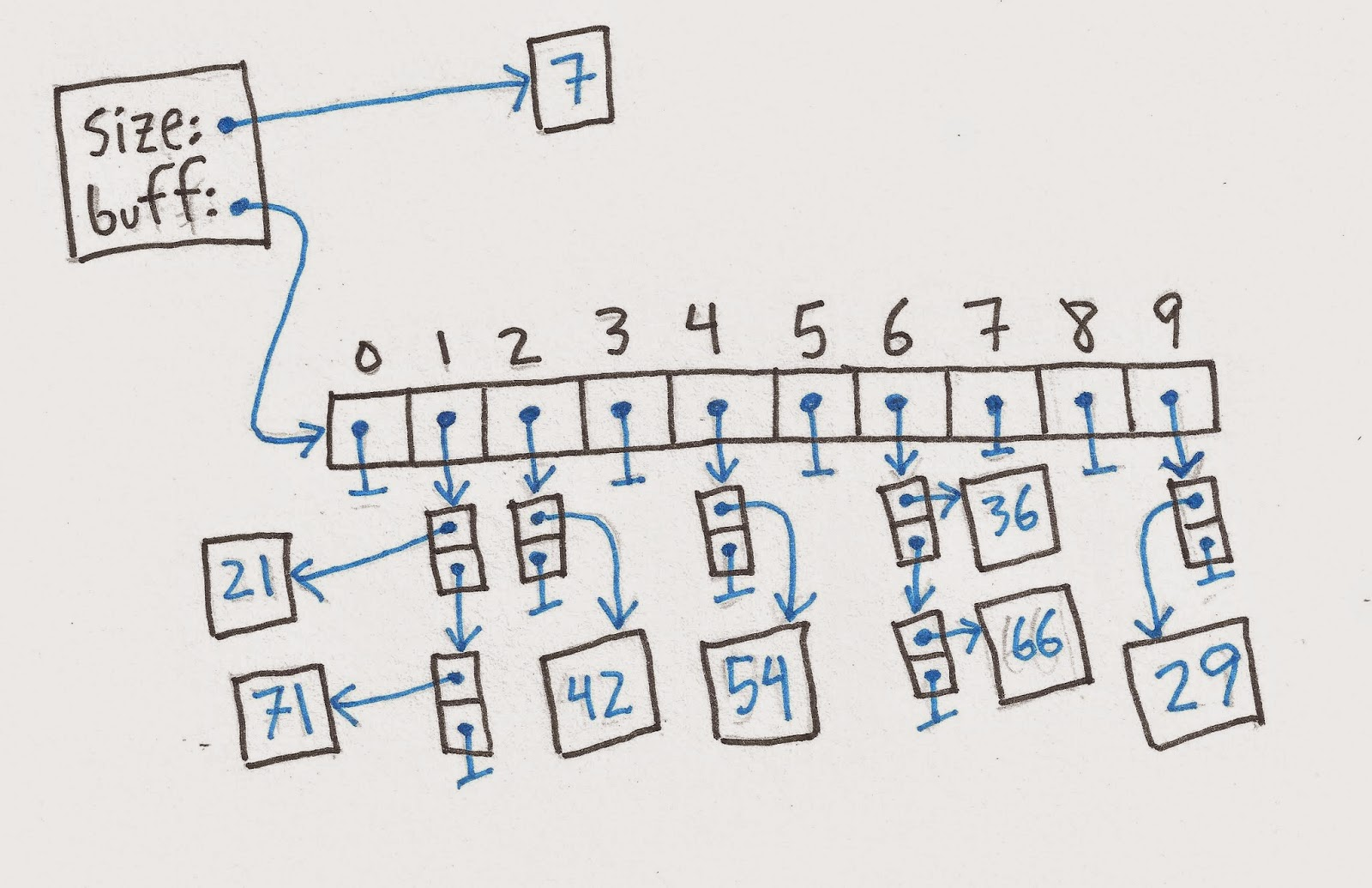Figure 8: Hash Set with Size 7
You will notice that two of the bucket lists are getting a little long, with two elements each. If these were to continue to grow, we would lose the O(1) behavior of most of the basic set operations.

To check for the existence of an element in the set, we determine the right bucket, and walk through the linked list looking for an element for which equals returns true. To remove an element from the set, we need to remove it from the appropriate linked list, which again requires walking the list. To add an element to the set, you might think we could avoid walking the list by inserting to the beginning of the list. But that doesn't quite work, as we need to avoid adding duplicate elements. We still have to walk the list, comparing the new element with existing elements using equals.

Hash sets perform extremely well compared to other set implementations. But this performance depends on the bucket lists being very short - ideally O(1). At least two factors are required to keep these lists short. First, the hashCode method of the elements in the set needs to avoid assigning the same or similar hash codes to different objects. Second, we need to expand the size of the array as the size of the set grows. When we expand, (or contract), we need to rebuild the entire hash table, since all existing elements will be assigned to a new bucket. We refer to rebuilding the hash table in this manner as rehashing.

When to expand the hash table is a matter of heuristics, and in some cases, (for example, Java's HashSet), is even configurable by the library user. Typically, we say that when the size of the set becomes greater than some fraction of the size of the array, it's time to expand. This fraction is called the load factor, and is commonly set to 75%. So in our example from figure 8, if we add one more element to the set, it's time to expand. Here's what the set will look like after the completion of an add(98) operation:Figure 9: Hash Set with Size 8
We doubled the size of our buffer, and rehashed every element into a new bucket. To find the new bucket, we use the hashCode modulo 20, instead of the hashCode modulo 10 that we used with the smaller buffer. Now, none of the linked lists contain more than a single element.

Rehashing is an O(n) operation for two reasons, just as with expanding and contracting the buffer with our ArrayBuffer example. First, allocating the new array is an O(n) operation in itself. Second, every element of the set needs to be placed in a new bucket. This second step is the equivalent of our use of Array.copy in ArrayBuffer.

Conclusions

By this point, we all should have a good understanding of just what amortized constant time actually means. We've looked at two examples of collections with amortized constant time operations. We've examined the performance characteristics of these operations both with charts, and with mathematics. And we've looked in detail at the code for one of these collections. All the code presented in this article is available in a GitHub project. Please feel free to download and play around with it. The unit tests there are kind of scant, so if you write any good tests, please submit a pull request!

I'm looking forward to investigating effective constant time with you in a future blog post!

I've put together a handful of questions for the reader while I was writing this article. If you like puzzles, or you want to self-test your understanding of the material, give one or more of them a try. If you're not interested, don't bother! Please try to avoid posting comments that might give away the answers. Thanks!

1. Under what kinds of circumstances might an amortized constant time operation not be a sufficient equivalent to a constant time operation?

2. Show that if an operation is linear time, then it is also amortized linear time. (Hint: use the definitions in equations 2 and 7.)

3. What if we expanded the array buffer by a factor of 1.5 instead of 2? Would we still get amortized constant time? Look over the math in equation 11. What would have to change if we changed the ExpansionRatio?

4. Suppose that we have a function f(n), and we also have upper bounds for the averages of f(n) for three series of input values:

Show that the complete series has the same upper bound:

Can you generalize this result for k averages all bounded by the same constant?

5. We have seen how amortized constant time operations vary between O(1) and O(n) behavior, depending on the input size. Because the O(n) operations were interspersed with O(1) operations at a rate of 2-n, the average case time complexity was O(1). Could the O(n) operations occur more frequently while still maintaining amortized constant time? What if the O(n) operations were interspersed at a rate of n-2?

6. What if our algorithm interspersed O(n2) operations among the O(1) operations? How infrequently would the O(n2) operations have to occur to get amortized constant time?

1 comment:

1.I am not very good in complexity analysis. I was solving a question on amortized complexity concept. I read this article and I am feeling good. You have explained it properly but due to my lack of comprehension I was unable to grab the last quarter of the article.